Next: Call external objects Up: Elements of the Fib Previous: if   Contents   Index

## Conditions with the if-element

To evalue subobjects depending on variables, the if-element can be used.

Syntax: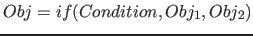If the condition is true, the first subobject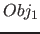will be evalued, else, if the condition is false, the second subobjectwill be evalued.

The following conditions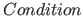are available (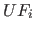are subfunctions as described for function element in section 9.7 on page):

•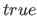: the condintion is true
•: the condintion is false (=not true)
•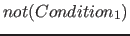: the condintion is true, if the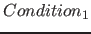is false (respectively not true), else the condition is false
•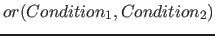: the condintion is true, if one or more of the conditionsorare true, else the condition is false
•: the condintion is true, if both of the conditionsandare true, else the condition is false
•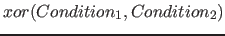: the condintion is true, if exactly one of the conditionsoris true, else the condition is false

•: the condintion is true, if the rounded to an integer value of the subfunction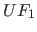is equal to the rounded to an integer value of the subfunction, else the condition is false; The direct comparison of floating point numbers was rejected, because due to slightly rounding errors the condition can lead to different results on different systems.
•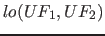: the condintion is true, ifis smaler than(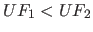), else the condition is false
•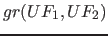: the condintion is true, ifis greater than(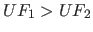), else the condition is false

Notes: Theconstruct is one of the most powerful programming language constructs, so it was included in the Fib multimedia description language.

Examples:

•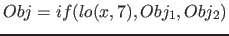;will be evalued, if the variableis smaler than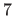, else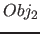will be evalued
•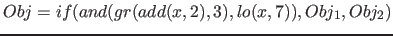;will be evalued, if the variableplusis greater thanandis smaler than(thus ifis a number betweanand), elsewill be evaluedNext: Call external objects Up: Elements of the Fib Previous: if   Contents   Index
Betti Österholz 2013-02-13